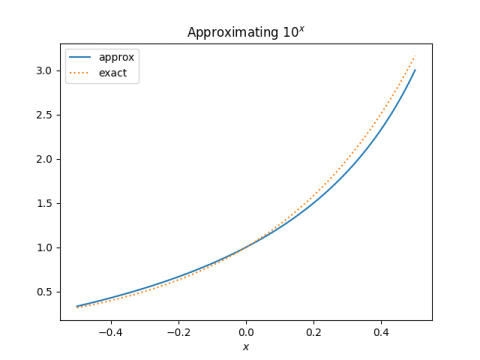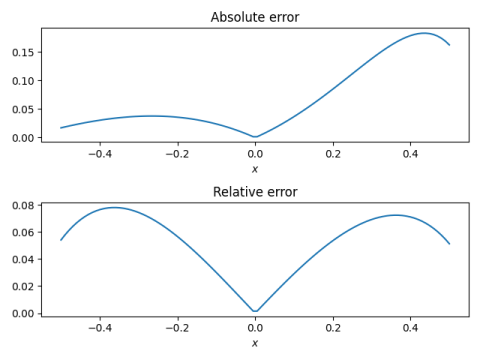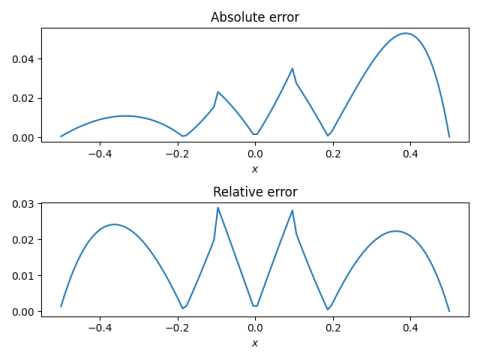# Mentally calculating 10^x

This is the last in a sequence of three posts on mental calculation. The first looked at computing sine, cosine, and tangent in degrees. The second looked at computing logarithms, initially in base 10 but bootstrapping from there to other bases as well.

In the previous post, we showed that

log10 x ≈ (x-1)/(x+1)

for x between 0.3 and 3.

If we take the inverse of the functions on both sides we get

10x ≈ (1+x)/(1-x).

for x between -0.5 and 0.5.

If the fractional part of x is not between -0.5 and 0.5 use

10x + 0.5 = 10x 100.5

to reduce it to that case.

For example, suppose you want a rough estimate of 102.7. Then

102.7 = 102 100.5 100.2 ≈ 100 × 3 × 1.2/0.8 = 450

This calculation approximates the square root of 10 as 3. Obviously you’ll get a better answer if you use a better approximation for square root of 10. Incidentally, π is not a bad approximation to √10; using 3.14 would be better than using 3.

## Plots and error

The graph below shows how good our approximation is. You can see that the error increases with x.But the function we’re approximating also grows with x, and the relative error is nearly symmetric about zero.Relative error is more important than absolute error if you’re going to multiply the result by something else, as we do when the fractional part of x has absolute value greater than 0.5.

## Improvement

The virtue of the approximation above is that it is simple, and moderately accurate, with relative error less than 8%. If you want more accuracy, but still want something easy enough to calculate manually, a couple tweaks make the approximation more accurate.

The approximation

10x ≈ (1+x)/(1-x)

is a little high on the left of zero, and a little low on the right. You can do a little better by using

10x ≈ 0.95 (1+x)/(1-x)

for -0.5 < x < -0.1, and

10x ≈ 1.05 (1+x)/(1-x)

for 0.1 < x < 0.5.Now the relative error stays below 0.03. The absolute error is a little high on the right end, but we’ve optimized for relative error.

In case you’re wondering where the factors of 0.95 and 1.05 came from, they’re approximately 3/√10 and √10/3 respectively.

Update: The approximations from this post and several similar posts are all consolidated here.

## 2 thoughts on “Mentally calculating 10^x”

1. Inverting the functions on both sides of an equation (an approximation in this case) is a technique I’ve not seen before. Does it have a name?

2. Diethard Michaelis

10^.5 is better approximated by 19/6 (.3% error) than by 22/7 or pi.
And 10^.3 is nicely approximated by 2 (.3% error) which gives easy approximations for 10^.6 and 10^.9 as well.